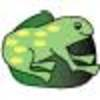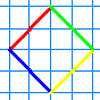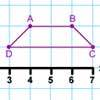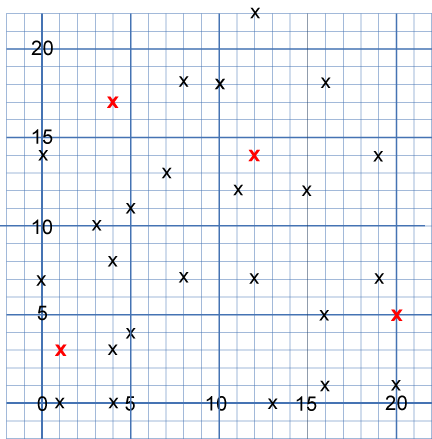#### You may also like### The Lily Pond

Freddie Frog visits as many of the leaves as he can on the way to see Sammy Snail but only visits each lily leaf once. Which is the best way for him to go?### A Cartesian Puzzle

Find the missing coordinates which will form these eight quadrilaterals. These coordinates themselves will then form a shape with rotational and line symmetry.### Transformation Tease

What are the coordinates of this shape after it has been transformed in the ways described? Compare these with the original coordinates. What do you notice about the numbers?

# Eight Hidden Squares

## Eight Hidden Squares

On the graph below there are $28$ marked points.These points all mark the vertices (corners) of eight hidden squares.
Each of the $6$ red points is a vertex shared by two squares.
The other $24$ points are each a vertex of just one square.
All of the squares share just one vertex with another square.
All the squares are different sizes.
There are no marked points on the sides of any square, only at the vertices.

Can you find the eight hidden squares?

### Why do this problem?

This problem has two aspects. A secure knowledge of the properties of squares is required and also acquaintance with coordinates in the first quadrant.

### Possible approach

This printable worksheet may be useful: Eight Hidden Squares.

You could start by drawing a square in various orientations, each time asking what shape you have drawn. Often children will say "diamond" when a square is presented diagonally. Alternatively, if the group is already familiar with squares in different orientations, you could start by drawing some shapes by giving their coordinates. This sheet could be used on an interactive whiteboard or enlarged to A3 and drawn on directly.

You could continue by choosing one point on the grid of the actual problem and then selecting a second point to form a second corner of a square. You could then ask where a third corner could be now and if there isn't a cross in the position, ask the class to suggest alternatives. Once you have found a possible third corner, ask where the fourth corner would have to be in order to make a square.

After this introduction children could work in pairs on the rest of the problem so that they are able to talk through their ideas with a partner. They could use this sheet for rough work and for recording.

At the end it would be useful to discuss the different orientations and sizes of the eight hidden squares as well as collecting together their coordinates.

Students could then go on to play the game Square It.

### Key questions

How does that square lie on the grid?
Where could its other vertices be?
Can you find another vertex for it?

### Possible extension

Students who are familiar with coordinates in all four quadrants could try the similar problem Ten Hidden Squares instead.
A suitable follow up problem is Square Coordinates, which encourages exploration of the relationship between coordinates of the vertices of squares.

### Possible support

Suggest using this sheet and sketching out possible squares one at a time starting with one point and looking for others that make a square with it. It might be helpful for some children to try Complete the Square first.